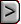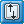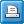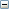﻿ PES - Parameter-Estimation Process Input File
Online Guide to MODFLOW

PES - Parameter-Estimation Process Input FilePES - Parameter-Estimation Process Input FilePES - Parameter-Estimation Process Input FileAbbreviation in Name file

PES

Purpose

The Parameter-Estimation Process is used to calibrate a model by modifying parameter values until the fit between the simulated values and observations is optimal.

Documentation

Hill, M.C., Banta, E.R., Harbaugh, A.W., and Anderman, E.R., 2000, MODFLOW-2000, the U.S. Geological Survey modular ground-water model -- User guide to the Observation, Sensitivity, and Parameter-Estimation Processes and three post-processing programs: U.S. Geological Survey Open-File Report 00-184, 210 p.

Supported in

MODFLOW-2000

Other Notes

 • The Parameter-Estimation Process requires that the flow, observation, and sensitivity processes all be used.
 • See Package Incompatibilities
 • The Parameter Estimation Process is not included in MODFLOW-2005 or MODFLOW-LGR.  External programs such as UCODE must be used instead.

Input Instructions

Input for the Parameter-Estimation Process is read from a file that is specified with file type "PES" in the name file.

Data Set 0

[#Text]

Item 0 is optional and can include as many lines as desired. Each line needs to begin with

the “#” character in the first column.Text
 Text—is a character string (maximum of 79 characters) that starts in column 2. Any characters can be included in Text. The “#” character needs to be in column 1. Text is printed when the file is read and provides an opportunity for the user to include information about the model both in the input file and the associated output file.

Data Set 1

MAX-ITER MAX-CHANGE TOL SOSC (free format)

Item 1 includes variables that are most often changed by the user.Explanation of variablesMAX-ITER
 MAX-ITER—is the maximum number of parameter-estimation iterations. If MAX-ITER = 0, the program calculates the variance-covariance matrix on parameters and related statistics (the parameter correlation coefficients generally are of most interest) using the starting parameter values from the Sensitivity Process input file, and parameter estimation stops after one iteration.MAX-CHANGE
 MAX-CHANGE—is the maximum fractional change for parameter values in one iteration (Hill, 1998, eq. 5, p. 9). MAX-CHANGE commonly equals 2.0, or less if parameter values are unstable during parameter-estimation iterations.TOL
 TOL—is the parameter-estimation closure criteria, as a fractional change in parameter values (Hill, 1998, eq. 7, p.12). TOL commonly equals 0.01. Larger values often are used during preliminary calibration efforts; values as small as 0.001 may be used for theoretical work.SOSC
 SOSC—is the second convergence criterion discussed in Hill (1998, p. 12). If SOSC ≠ 0.0, parameter estimation will converge if the least-squares objective function does not decrease by more than SOSC*100 percent over two parameter-estimation iterations. SOSC usually equals 0.0. Typical nonzero values of SOSC are 0.01 and 0.05.

Data Set 2

IBEFLG IYCFLG IOSTAR NOPT NFIT SOSR  RMAR RMARM IAP (free format)

Item 2 includes variables that control the modified Gauss-Newton calculations and are sometimes changed by the user.Explanation of variablesIBEFLG

IBEFLG—is a flag that controls the generation of files to be used as input to the post-processing program BEALE-2000, which tests model linearity.

 • IBEFLG = 0, no file for BEALE-2000 is produced.
 • IBEFLG = 1, the _b1 file of tables 5 and 12 is produced.
 • IBEFLG = 2, the _b2 file of tables 5 and 13 is produced. Production of this file may require MODFLOW-2000 input files that differ from the files used for model calibration, as discussed in chapter 7.IYCFLG
 • IYCFLG—is a flag that controls the generation of files to be used as input to the post-processing program YCINT-2000, which calculates confidence and prediction intervals on simulated equivalents to observations.
 • IYCFLG = 0, The _y0 file of tables 5 and 9 is produced. Production of this file may require MODFLOW-2000 input files that differ from the files used for model calibration.
 • IYCFLG = 1, The _y1 file of tables 5 and 10 is produced. Production of this file may require MODFLOW-2000 input files that differ from the files used for model calibration, as discussed in chapter 7. Sensitivities for the predicted quantities are calculated, but the calculations related to nonlinear regression and the variance-covariance matrix on parameters are not made.
 • IYCFLG = 2, The _y2 file of tables 5 and 11 is produced. This file is needed if confidence and prediction intervals on differences (eq. 7) are to be calculated. Production of this file may require MODFLOW-2000 input files that differ from the files used for model calibration, as discussed in chapter 7. Sensitivities for the predicted quantities are calculated, but the calculations related to nonlinear regression and the variance-covariance matrix on parameters are not made.IOSTAR
 IOSTAR—is a flag that controls printing to the screen. If IOSTAR equals one, printing to the screen is suppressed. Usually IOSTAR=0.NOPT
 NOPT—is a flag identifying whether or not to include matrix R of equation (B1) in equation (4a), as described in Hill (1998, p. 8, 78). Regression may converge in fewer iterations with NOPT = 1 for problems with large residuals and a large degree of nonlinearity.NFIT
 NFIT—is the number of Gauss-Newton iterations (when NOPT equals 1) after which matrix R of equation (B1) is included in equation (4a) of Hill (1998, p. 8, 79).SOSR
 SOSR—is a criterion for using R of equation (B1) in equation (4a) of Hill (1998, p. 8,78). Matrix R is used if the percentage change in the sum of squared, weighted residuals does not exceed SOSR*100 in two parameter-estimation iterations. Usually SOSR equals 0.0.RMAR
 RMAR—is used along with RMARM to calculate the Marquardt parameter, if its use is indicated based on CSA of item 4. The calculation of the Marquardt parameter described by Hill (1998, p. 9) is expressed as mrnew= RMARM*mrold+ RMAR. Typically, RMAR = 0.001.RMARM
 RMARM—is used along with RMAR to calculate the Marquardt parameter, if its use is indicated based on CSA of item 4. The calculation of the Marquardt parameter described by Hill (1998, p. 9) is expressed as mrnew= RMARM*mrold+ RMAR. Typically, RMARM = 1.5.IAP

IAP—is a flag identifying whether, for log-transformed parameters, MAX-CHANGE applies to the native parameter value or to the log transform of the parameter value. Generally, IAP = 0.

 • IAP = 0, MAX-CHANGE applies to the native parameter value.
 • IAP = 1, MAX-CHANGE applies to the log transform of the parameter value.

Data Set 3

IPRCOV IPRINT LPRINT (free format)

Item 3 includes variables that control printing.Explanation of variablesIPRCOV

IPRCOV—is a format code for printing of variance-covariance and correlation matrices. Permissible values of IPRCOV and corresponding formats are:

 IPRCOV FORMAT 1 11G10.3 2 10G11.4 3 9G12.5 4 8G13.6 5 8G14.7 6 6G10.3 7 5G11.4 8 5G12.5 9 4G13.6 10 4G14.7IPRINT

IPRINT—is a flag that controls printing of various statistics computed for each parameter-estimation iteration, including simulated equivalents, unweighted and weighted residuals, observation sensitivities, summary statistics for residuals by observation type, scaled least-squares matrix of the Gauss-Newton method, and scaled gradient vector of the objective function.

 • IPRINT = 0, the statistics are printed at the first and last parameter-estimation iterations.
 • IPRINT > 0, the statistics are printed at each iteration. Also, a summary of parameter values and statistics for all parameter-estimation iterations is printed in theGLOBAL output file.LPRINT

LPRINT—is a flag that controls printing of eigenvalues and eigenvectors.

 • LPRINT = 0, eigenvalues and eigenvectors are not printed.
 • LPRINT > 0, if parameter estimation converges, eigenvalues and eigenvectors are printed.

Data Set 4

CSA FCONV LASTX (free format)

Item 4 includes variables that control the modified Gauss-Newton calculations and are rarely changed by the user.Explanation of variablesCSA
 CSA—is the search-direction adjustment parameter used in the Marquardt procedure. Usually equals 0.08.FCONV
 FCONV—is a flag and a value used to allow coarser solver convergence criteria for early parameter-estimation iterations. If FCONV equals zero, coarser convergence criteria are not used. Commonly, FCONV = 0.0; typical nonzero values would be 5.0 or 10.0, and these can produce much smaller execution times in some circumstances.LASTX

LASTX—is a flag that controls calculation of the sensitivities used to calculate the parameter variance-covariance matrix when parameter estimation converges.

 • LASTX = 0, sensitivities from the last parameter-estimation iteration are used to calculate the variance-covariance matrix. The program proceeds as in figure 1.
 • LASTX > 0, sensitivities are recalculated using the final parameter estimates and are used to calculate the variance-covariance matrix.

Data Set 5

NPNG IPR MPR (free format)

Item 5 includes variables that indicate whether additional items need to be read. The additional possible items are as follows.Explanation of variablesNPNG
 NPNG—is the number of parameters of type HK, VK, VANI, VKCB, SS, SY, EVT, or ETS that can have negative values. This is useful for some interpolation methods in which, for example, deviations from a base value are calculated, where the deviations can be positive or negative. An example of such a method is described by Keidser and Rosbjerg (1991). If NPNG is greater than zero, item 6 is read.IPR
 IPR—is the number of parameters included in the full variance-covariance matrix used to weight the prior information.MPR
 MPR—is the number of prior-information equations to be used in the regression.

Data Set 6

If NPNG is greater than zero, read item 6 once.

PARNEG(1), PARNEG(2), . . . , PARNEG(NPNG) (free format)PARNEG
 PARNEG—is an array of NPNG names of parameters of type HK, VK, VANI, VKCB, SS, SY, EVT, or ETS that can have negative values. This may be the case when the second kriging method discussed in Hill (1992, p. 125) is used.

Data Set 7

If IPR is greater than zero, read item IPR repetitions of item 7. Parameters that appear in item 7 may not appear in item 10.

NIPRNAM BPRI PLOT-SYMBOL (free format)Explanation of variablesNIPRNAM
 NIPRNAM—is the name of one of the IPR parameters for which a variance-covariance matrix for prior parameter estimates is to be read.BPRI
 BPRI—is the prior estimate for parameter NIPRNAM.PLOT-SYMBOL
 PLOT-SYMBOL—is an integer that will be written to output files intended for graphical analysis to allow control of the symbols used when plotting data related to the prior information.

If IPR is greater than zero, read items 8 and 9 once. The size of item 9 depends on IPR

Data Set 8

IWTP (free format)IWTP

IWTP—is a flag identifying how the weight matrix for correlated prior information is to be calculated using the values in array WTP specified in item 9.

 • IWTP = 0, WTP is a variance-covariance matrix. The diagonal terms of this matrix are the variances of the prior information, the off-diagonals are the covariances. Diagonal term WTP(I,I) is the variance for the parameter designated by NIPRNAM(I); off-diagonal term WTP(I,J) is the covariance for the parameters designated by NIPRNAM(I) and NIPRNAM(J). For parameters specified as being log-transformed in the Sensitivity Process input file, the corresponding WTP elements are interpreted as being relative to the log-transformed value (using log base 10). The weight matrix is calculated by taking the inverse of the array specified in item 9.
 • IWTP = 1, WTP is a matrix of coefficients of variation (the standard deviation divided by the prior information value) and correlation coefficients. The diagonal terms are the coefficients of variation of the prior information; off-diagonals are the correlation coefficients and vary in value from –1.0 to +1.0. Diagonal term WTP(I,I) is the coefficients of variation for the parameter designated by NIPRNAM(I); off-diagonal term WTP(I,J) is the correlation coefficients for the parameters designated by NIPRNAM(I) and NIPRNAM(J). For parameters specified as being log-transformed in the Sensitivity Process input file, the corresponding WTP elements are interpreted as being relative to the log-transformed value (using log base 10). The weight matrix is calculated by in two steps. First, the coefficients of variation and correlation coefficients are used to calculate variances and covariances, and then the inverse of the variance-covariance matrix is calculated. In calculating variances, the coefficients of variation are multiplied by the prior information values specified in item 7, or the log10 of that value for log-transformed parameters; if the value equals zero, 1.0 is used instead.

Data Set 9

WTP(1,1), WTP(1,2), ..., WTP(1,IPR) (free format)

WTP(2,1), WTP(2,2), ..., WTP(2,IPR) (new line; free format)

...

WTP(IPR,1), WTP(IPR,2), ..., WTP(IPR,IPR) (new line; free format)WTP
 WTP—is an IPR by IPR array containing statistics used to calculate the weight matrix for correlated prior information. The statistics specified depends on the value of IWTP. Note that the matrix is symmetric, but the entire matrix (upper and lower parts) must be entered.

Data Set 10

If MPR is greater than zero, read MPR repetitions of item 10. Examples are shown after the next section. Parameters that appear in item 7 may not appear in item 10.

EQNAM PRM "=" [SIGN] [COEF "*"] PNAM [SIGN [COEF "*"] PNAM [SIGN…]] "STAT" STATP STAT-FLAG PLOT-SYMBOL (free format: maximum of 200 characters; one or more spaces must separate all words, numbers, and symbols)Explanation of variablesEQNAM
 EQNAM—is a user-supplied name (up to 10 nonblank characters) for a prior-information equation.PRM
 PRM—is the prior estimate for prior-information equation EQNAM. PRM always needs to be specified as a native, untransformed value. That is, even if the parameter is specified as being log-transformed in the Sensitivity Process input file, here PRM needs to be the untransformed value. The program will calculate the log-transformed value."="
 "=" indicates that an equal sign (without quotes) must be entered literally.SIGN
 SIGN—is either "+" or "-" (entered without quotes). The SIGN before the first PARNAM is assumed to be “+” unless otherwise indicated.COEF
 COEF—is the coefficient for the parameter following the "*" in prior-information equation EQNAM. COEF can be specified with or without a decimal point and can be specified in scientific notation."*"
 "*"—indicates that an asterisk (without quotes) must be entered literally if a value for COEF is entered.PNAM
 PNAM—is a parameter name (up to 10 nonblank characters) as specified in the SEN file. If the parameter is designated in the Sensitivity Process input file as being log-transformed (LN greater than 0), the prior-information equation may contain only one parameter name. If a prior-information equation contains no log-transformed parameters, the equation may contain any number of terms, where each term is defined by the sequence: SIGN [COEF "*"] PNAM."STAT"
 "STAT"—indicates that the word “STAT” (without quotes) must be entered literally, although it may be in any combination of upper- and lowercase letters.STATP
 STATP—is the value from which the weight for prior-information equation EQNAM is calculated, as determined using STAT-FLAG. If a parameter is specified as being log-transformed in the Sensitivity Process input file, STATP may be specified relative either to the native value or to the log-transformed value (using log base 10), depending on the value of STAT-FLAG.STAT-FLAG

STAT-FLAG—is a flag identifying how the weight for prior-information equation EQNAM is to be calculated. This depends both on whether the user chooses to specify the variance, standard deviation, or coefficient of variation, and whether, for log-transformed parameters, the user chooses to specify the statistic related to the native, untransformed parameter, or to the transformed parameter.

 • STAT-FLAG = 0, STATP is the variance associated with PRM, and is related to the native prior value. Weight = 1/STATP unless the parameter is defined as log-transformed in the Sensitivity Process input file, in which case equation 27 is used to convert STATP (which equals σ2b of equation 27) to σ2ln b, and weight = 1/σ2ln b.
 • STAT-FLAG = 1, STATP is the standard deviation associated with PRM, and is related to the native prior value. Weight = 1/STATP2 unless the parameter value is defined as log-transformed in the Sensitivity Process input file, in which case equation 27 is used to convert STATP (which equals σb of equation 27) to σ2ln b, and weight = 1/σ2ln b.
 • STAT-FLAG = 2, STATP is the coefficient of variation associated with PRM, and is related to the native prior value. Weight = 1/(STATP * PRM)2 unless the parameter is defined as log-transformed in the Sensitivity Process input file, in which case equation 27 is used to convert STATP (which equals σb/b of equation 27), to σ2ln b, and weight = 1/σ2ln b.
 • STAT-FLAG = 10, STATP is the variance associated with the log (base 10) transform of PRM; weight = 1/[STATP * 2.30262].
 • STAT-FLAG = 11, STATP is the standard deviation associated with the log (base 10) transform of PRM; weight = 1/[STATP2* 2.30262].
 • STAT-FLAG = 12, STATP is the coefficient of variation associated with the log (base 10) transform of PRM; weight = 1/[(STATP * log 10 (PRM))2* 2.30262].PLOT-SYMBOL
 PLOT-SYMBOL—is an integer that will be written to output files intended for graphical analysis to allow control of the symbols used when plotting data related to the prior information.

Example Input File

# Example PES file

#

15 2.0 1E-5 1E-4 ITEM 1: MAX-ITER MAX-CHANGE TOL SOSC

0 0 0 0 0 0. .001 1.5 0 ITEM 2: IBEFLG IYCFLG IOSTAR NOPT NFIT SOSR RMAR RMARM IAP

2 0 0 ITEM 3: IPRCOV IPRINT LPRINT

0.08 0.0 0 ITEM 4: CSA FCONV LASTX

0 0 6 ITEM 5: NPNG IPR MPR

EQ-Q_3 -97000. = WQ_3 STAT 1940 1 9 ITEMS 10: 6 (MPR) PRIOR-INFO EQUATIONS

EQ-Q_4 -51000. = WQ_4 STAT 1020 1 9

EQ-HK_2 420. = HK_2 STAT 84. 1 9

EQ-RCH_1 0.0004 = RCH_ZONE_1 stat 1.2E-4 1 9

EQ-RCH_3 1.7E-4 = RCH_ZONE_3 stat 5.1E-5 1 9

EQ-KRB_1 0.08 = RIVERS stat 0.008 1 9

In this example, WQ_3, WQ_4, HK_2, RCH_ZONE_1, RCH_ZONE_3, and RIVERS are

parameter names, which need to be defined in input files for Groundwater Flow Process

packages and need to be listed with ISENS > 0 in the Sensitivity Process input file. The program

matches parameter names among the various input files in a case-insensitive manner. EQ-Q_3,

EQ-Q_4, EQ-HK_2, EQ-RCH_1, EQ-RCH_3, and EQ-KRB_1 are names assigned to the 6 (MPR)

prior-information equations.